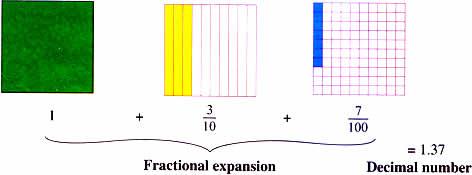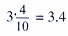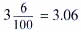Friday , September 18 2020# NCERT 5th Class (CBSE) Mathematics: Decimals

The place value of a digit decreases every time it move to the right. It gets ten times smaller for every move to the right.

The Decimals point is always placed after the ones place.

After the decimal point, the place values are tenths, hundredths, thousandths, … All these places have a value less than one.### Mathematical Model of Decimals#### How to read a decimal number?

1.37 is read as one thirty-seven hundredths. It is also read as one point three seven.Three and four tenths or three point four.

4/10 = 0.4

Four tenths or zero point fourThree and six hundredths or three point zero six

6/100 = 0.06

Six hundredths or zero point zero six.

## गुरु और चेला 5 NCERT CBSE Hindi Book Rimjhim Chapter 12

गुरु और चेला 5th Class NCERT CBSE Hindi Book Rimjhim Chapter 12 गुरु और चेला – प्रश्न: …